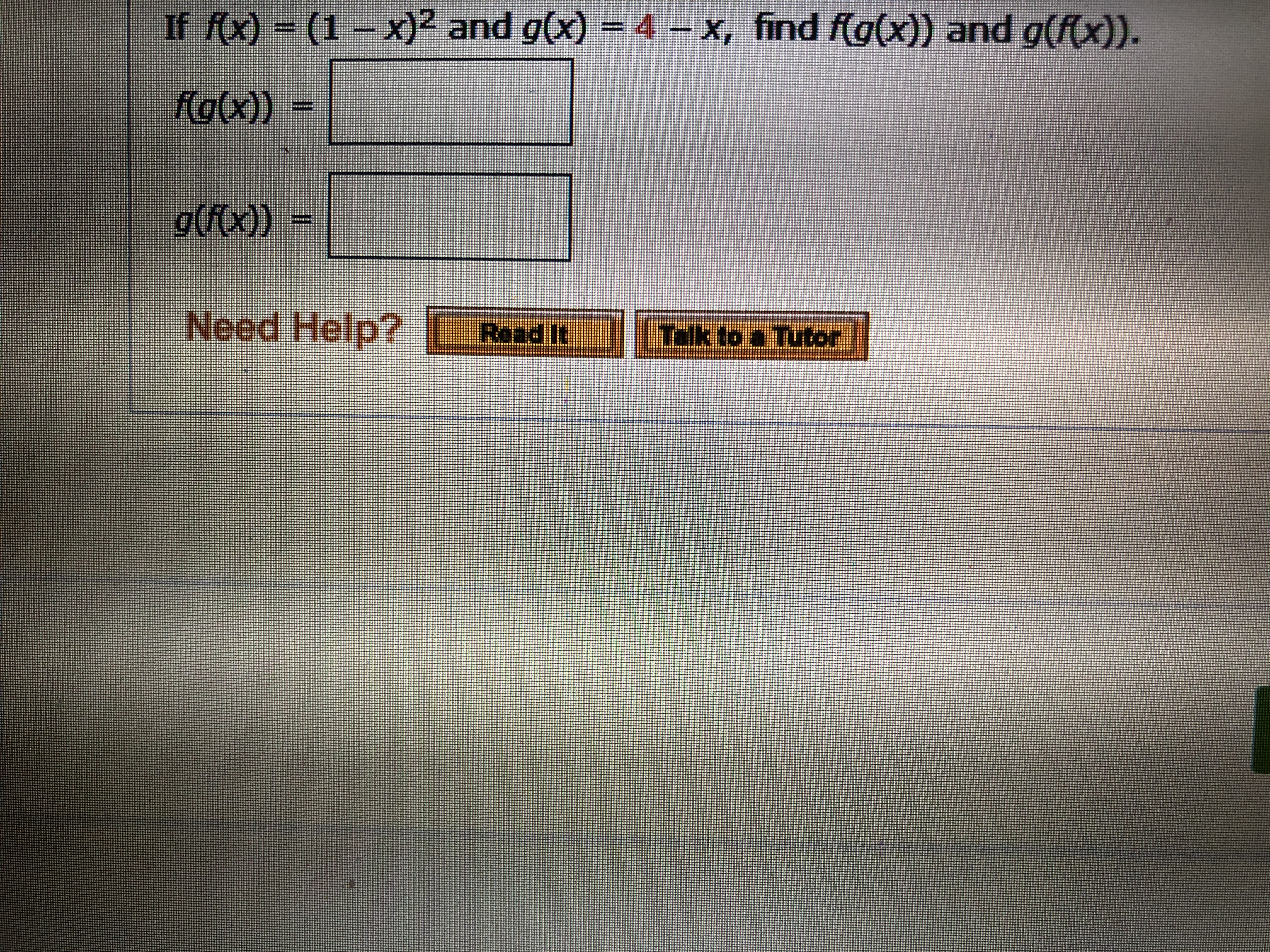# If fx) (1-x)2 and g(x) = 4-x, find f(g(x)) and g((x)). fo(x)) g((x)) Need Help? L Read It Tkto Tutor

Question

what are the blankshelp_outlineImage TranscriptioncloseIf fx) (1-x)2 and g(x) = 4-x, find f(g(x)) and g((x)). fo(x)) g((x)) Need Help? L Read It Tkto Tutor fullscreen

### Want to see the step-by-step answer?